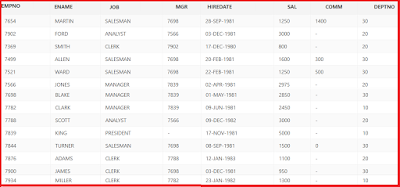# Aggregate and Group Functions in SQL and Oracle with examples

• Aggregate functions will acts group of values at a time and returns a single value. It is also called Group functions.
• Aggregate functions will ignore NULL values while calculating.
• Here is the sample emp table using for solving examples.Emp Sample Table
1. Sum:
Sum is used to find the sum of values in a column.

Example:Write a query for find the total salary of emp table.
`select sum(sal) from emp`
2. Avg: Avg is used to find average values for given values.

Example:Write a query for find the average salary of emp table.

`select avg(sal) from emp`

3. Max: Max will find the maximum value from given values.

Example:Write a query for find the highest salary of emp table.

`select max(sal) from emp`

4. Min:Min will find the minimum value from given values.

Example:Write a query for find the lowest salary of emp table.

`select min(sal) from emp`

5. Count: count will count the number of values in a group.

It will take 3 different parameters

a. count(*):Count the number of values in a group.

Example:

```select count(*) from emp

It will return 14```
b. count(column_name): It will count not null values in a column.

Example:
```select count(mgr) from emp

It will return 13 as KING ename won't be having any manager number,
it is NULL hence it will neglect the NULL value. ```

c. count (distinct column_name); It will count all not null values but ignore duplicate values.

Example:

```select count(distinct (mgr)) from emp

It will return 6 as it will removed duplicates as total
6 members are managers.```
Example: Write a query to find average sal and sum sal of emp.
```select sum(sal), avg(sal)
from emp```

#### ## Group By: It is used to divide the table into groups.

For example in emp table with 14 records, all managers are one group and all analysts are in another group etc.

Syntax:
select (column_list) group functions from
```(table_name) [where condition] group by
group by expression
[order by column or group function]
```
Example: Write a query to divide the table into groups based on deptno.
```select deptno, sum(sal), min(sal), max(sal)
from emp
group by deptno```
Example: Write a query to divide the table into groups for departments 10 and 30 and sort by total salary.
```select deptno, sum(sal), min(sal), max(sal)
from emp
where deptno in (10,30)
group by deptno
order by sum (sal)```

#### ## Having Clause: Having clause is useful to impose condition on the grouped data. where as WHERE condition is used to retrieve data based on the conditions of single data.

Question: Is it possible to use WHERE and HAVING in one statement?
```select (column_list) group functions from
(table_name) [where condition] group by
group by expression
having condition
[order by column or group function]
```
Example:Write a Query to divide the table into groups based on job and deptno anddisplay only clerk, manager and salesman group if no of employees in group are more than one.
```select job,deptno, sum(sal), min(sal), max(sal), count(*)
from emp
where job in ('CLERK','MANAGER','SALESMAN')
group by job, deptno
having count(*)>1```
Example:Write a Query to divide the table into groups based on job and display only analyst, manager and president group if no of total salary is more than 4000 and display the data in descending order of the total salary
```select job, sum(sal), min(sal), max(sal), count(*)
from emp
where job in ('ANALYST','MANAGER','PRESIDENT')
group by job
having sum(sal) > 4000<
order by sum(sal) desc```